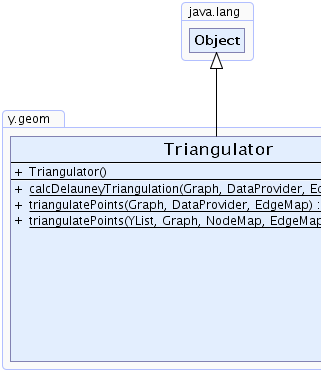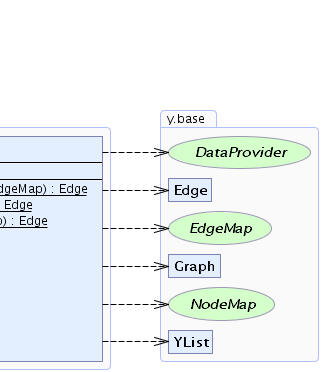Search this API

## y.geom Class Triangulator

```java.lang.Objecty.geom.Triangulator
```

`public class Triangulatorextends java.lang.Object`

This class provides algorithms for the triangulation of point sets in the plane.Constructor Summary
`Triangulator()`

Method Summary
`static Edge` ```calcDelauneyTriangulation(Graph result, DataProvider pointData, EdgeMap revMap)```
Computes a Delauney triangulation of the given points.
`static Edge` ```triangulatePoints(Graph result, DataProvider pointData, EdgeMap reverseEdgeMap)```
Computes a triangulation of the given points.
`static Edge` ```triangulatePoints(YList points, Graph result, NodeMap resultMap, EdgeMap reverseEdgeMap)```
Computes a triangulation of the given points.

Methods inherited from class java.lang.Object
`clone, equals, finalize, getClass, hashCode, notify, notifyAll, toString, wait, wait, wait`

Constructor Detail

### Triangulator

`public Triangulator()`
Method Detail

### triangulatePoints

```public static Edge triangulatePoints(Graph result,
DataProvider pointData,
EdgeMap reverseEdgeMap)```
Computes a triangulation of the given points. The calculated triangulation is represented by an embedded graph, i.e. to each edge there exists a reverse edge and the outedges around each node are in embedded order. The returned edge and the (optional) reverseEdgeMap can be used to construct all faces of the plane graph and to determine its outer face.

Parameters:
`result` - a graph whose nodes represent the points that need to be triangulated.
`pointData` - must provide the location (YPoint) for each node in the given graph.
`reverseEdgeMap` - a node map that will contain for each edge its reverse edge. If this argument is `null` then no reverse edge information will be available.
Returns:
an edge on the outer face of the result graph.

### triangulatePoints

```public static Edge triangulatePoints(YList points,
Graph result,
NodeMap resultMap,
EdgeMap reverseEdgeMap)```
Computes a triangulation of the given points. The calculated triangulation is represented by an embedded graph, i.e. to each edge there exists a reverse edge and the outedges around each node are in embedded order. The returned edge and the (optional) reverseEdgeMap can be used to construct all faces of the plane graph and to determine its outer face.

Parameters:
`points` - the point set to be triangulated. The points must be provided as a YList of YPoints.
`result` - the resulting triangulation
`resultMap` - the node map that forms the link between a point and a node.
`reverseEdgeMap` - a node map that will contain for each edge its reverse edge. If this argument is `null` then no reverse edge information will be available.
Returns:
an edge on the outer face of the result graph.

### calcDelauneyTriangulation

```public static Edge calcDelauneyTriangulation(Graph result,
DataProvider pointData,
EdgeMap revMap)```
Computes a Delauney triangulation of the given points. A Delauney triangulation is a triangulation such that none of the given points is inside the circumcircle of any of the calculated triangles.

The calculated triangulation is represented by an embedded graph, i.e. to each edge there exists a reverse edge and the outedges around each node are in embedded order. The returned edge and the (optional) reverseEdgeMap can be used to construct all faces of the plane graph and to determine its outer face.

Parameters:
`result` - a graph whose nodes represent the points that need to be triangulated.
`pointData` - must provide the location (YPoint) for each node in the given graph.
`revMap` - a node map that will contain for each edge its reverse edge. If this argument is `null` then no reverse edge information will be available.
Returns:
an edge on the outer face of the result graph.